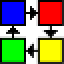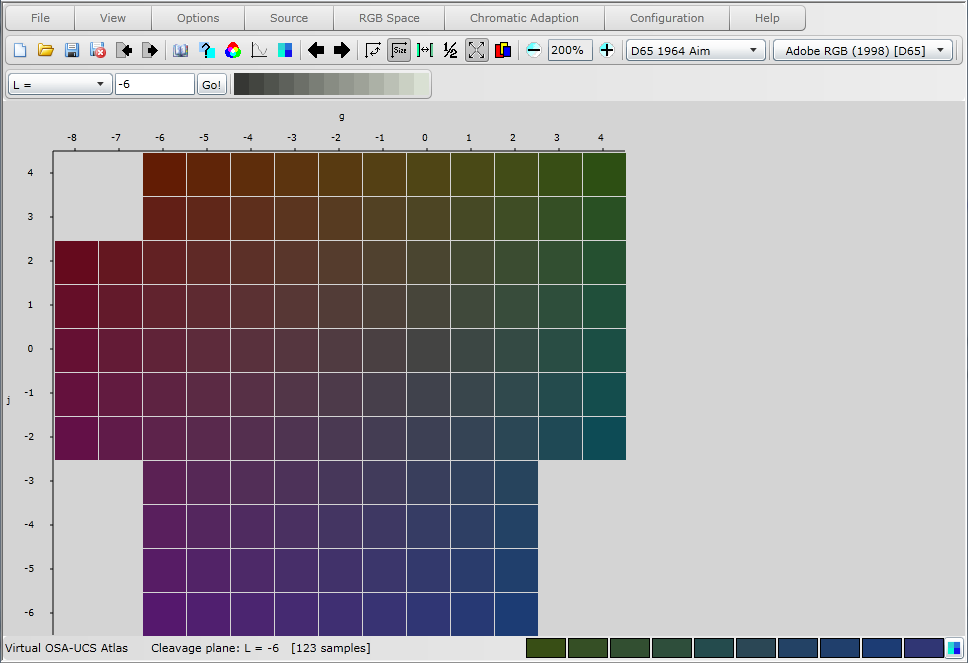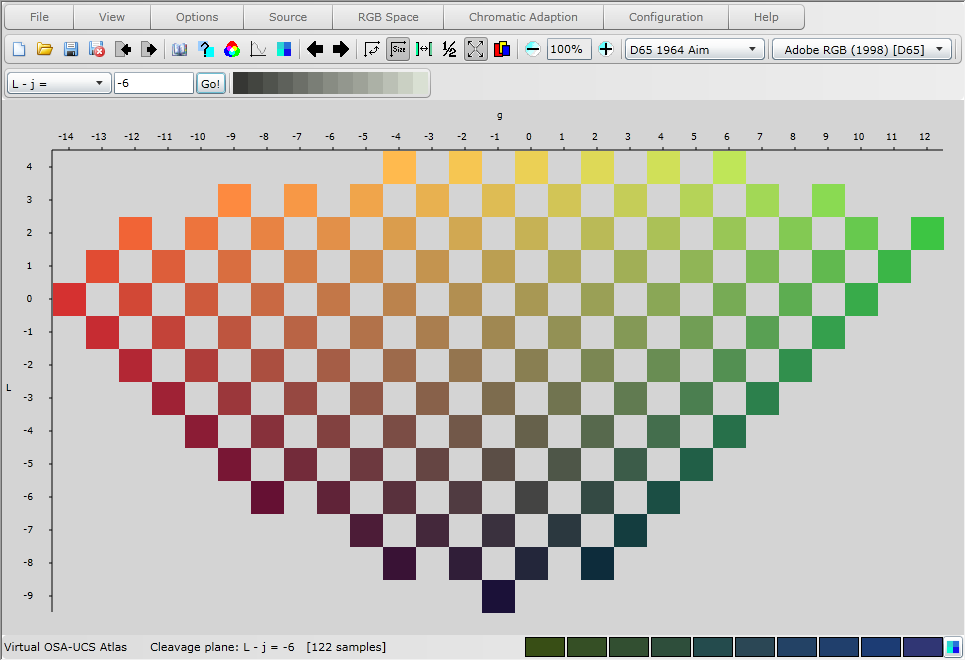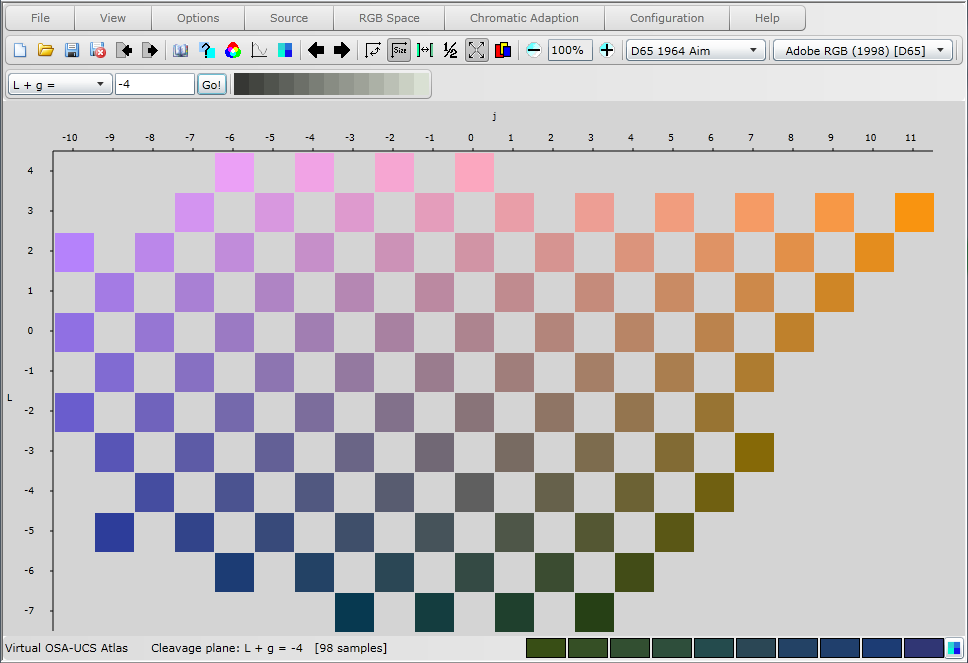Virtual Colour Systems Ltd. Virtual Atlas Home Help Menu
 OSA-UCS (Optical Society of America - Uniform Color Scales) The OSA-UCS system is unique in that it exemplifies uniform colour space by a regular rhombohedral lattice of colour samples. Each lattice point in OSA-UCS colour space is surrounded by twelve other lattice points (excepting a few limit points) each of which are equally perceptually different from it. This ensures that visual equality is achieved in all three dimensions of colour space. Each of these twelve points can also become a centre point and by this method the lattice is extended to encompass the whole of colour space. The arrangement of the points is best described as a cuboctahedron, with the twelve nearest neighbours situated at the vertexes surrounding the lattice point in the centre of the arrangement. A cuboctahedron is formed by cutting away the eight corners of a cube which form four pairs of triangular faces known as the octahedral faces. The cuboctahedron thus comprises four pairs of triangular faces and three pairs of square faces: the remains of the original cube. The lattice is constructed such that one set of square faces lie on the horizontal plane. The coloured samples on each horizontal plane display constant lightness. The lightness axis, L, runs vertically through OSA-UCS colour space, and is unique in that lightness is related to modified luminance to counteract the effect of chromaticity on lightness. The central plane represents the background lightness which is a medium grey corresponding to 30% reflectance and specified as Munsell value 6/. The central plane has an OSA lightness of L = 0. Planes above take increasing values of L in steps of equal lightness towards absolute white. Planes below are in steps of decreasing values of L towards absolute black.  Below is the constant lightness plane L = -6.In each constant lightness plane the OSA coordinates j and g are used to identify the individual lattice points; the L, j and g axes being perpendicular to each other. The OSA j coordinate takes its name from jaune, the French word for yellow (y was not used so as to avoid confusion with the CIE y chromaticity coordinate), and represents the yellowness-blueness axis. Positive values of j, with g = 0, indicate yellowish-brownish colours whilst negative values of j indicate bluish colours. The OSA g coordinate takes its name from green and represents the greenness-redness axis. Positive values of g, with j = 0, indicate greenish colours whilst negative values of g indicate reddish-purplish colours. Neutrals are expressed as varying degrees of L with j and g equal to 0; near-neutrals by small absolute values of both j and g.  The cleavage plane L - j = -6 is shown below.The complete OSA-UCS notation to uniquely identify colours is thus L,j,g. The following cleavage planes are supported: L =, j =, g =, L + j =, L - j =, L + g =, L - g =, j + g =, j - g =, j - L =, g - j =, and g - L =.  The cleavage plane L + g = 4 is shown below.Copyright 2011, Virtual Colour Systems Ltd. All Rights Reserved